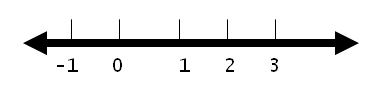Home Math Calculators Math  Flashcards Math Games Math  Homework Math Lessons Math Terms Math Worksheets Math Tools
You Are In Math Glossary of Terms

# Number Line

A number line is a line in which real numbers can be placed, according to their value.

Each point on a number line corresponds to a real number, and each real number has a unique point that corresponds to it.For example, the number 1 corresponds to the point on a number line that is halfway between zero and two.